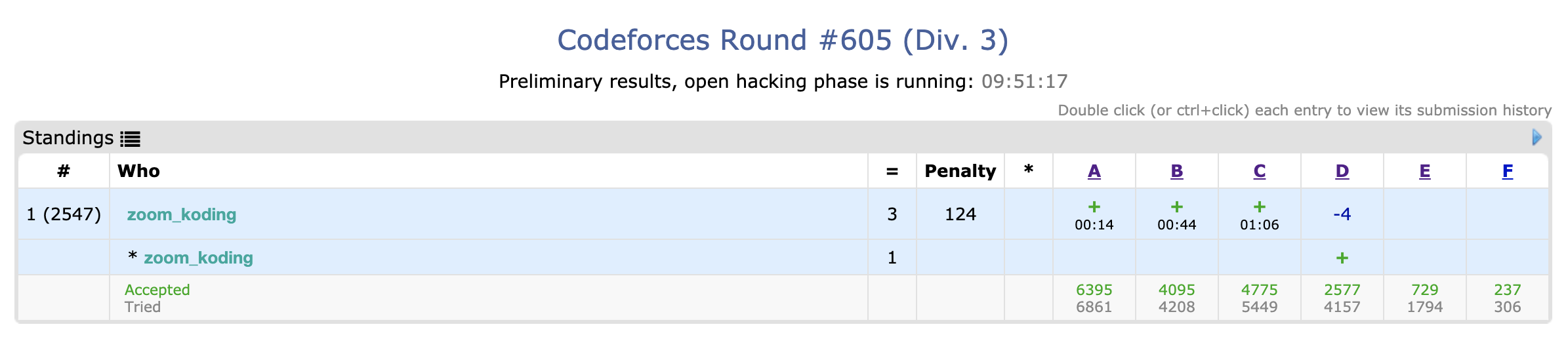## This Round

• Sucks… I solved first three problems in about an hour but stuck in problem D.
• Good Problems though!## A. Three Friends

At first, I was thinking of checking all the number of cases. However, I found a way to solve by sorting to find the first, middle and the last friend.

If two of them are staying together, the all of them moves to get closer. For example, 1, 99, 99 move to 2, 98, 98.

Otherwise, the middle one doesn’t have to move and the other two get closer to the middle one. For example, 1, 2, 5 move to 2, 2, 4.

d1, d2 represent the distances of the friends.

### Code

#include <cstdio>
#include <algorithm>
using namespace std;
long long n, arr;
int main(){
scanf("%lld", &n);
while(n--){
scanf("%lld %lld %lld", &arr, &arr, &arr);
sort(arr, arr+3);
long long d1 = arr - arr, d2 = arr - arr;
if(d1 == 0 && d2 == 0){
printf("0\n");
continue;
}
if(d1 == 0){
d2 -= 2;
if(d2 < 0)d2 = 0;
}
else if(d2 == 0){
d1 -= 2;
if(d1 < 0) d1 = 0;
}
else d1 --, d2 --;
printf("%lld\n", (d1 + d2) * 2);
}
}


## B. Snow Walking Robot

If you appoached this problem by checking whether a move is valid, it would be very complex. I think the key of this question is that the order of the remaining maximum moves can be changable.

I first receive all the numbers of RLUD and use them to find maximum number. I call minRL, the smaller count of R and L, and minUD, the smaller count of U and D. If minRL and minUD are not zero, we can make a cycle to come back. There are three ways to maximize the remaining moves.

• If minRL is 0 and minUD is 0, the answer is 0.
• Ex) LLL => 0
• If one of minRL and minUD is 0, the answer is 2.
• Ex) RRRRLLLL => 2, RL
• Otherwise, the answer is (minRL + minUD) * 2 and print the result in the order of RULD to avoid visiting the same position twice.
• Ex) URRDURLL => 6, RRULLD

### Code

#include <cstdio>
#include <algorithm>
using namespace std;
int t, arr, minRL, minUD, ans;
char s;
int main(){
scanf("%d", &t);
while(t--){
int cnt = 0;
scanf("%s", s);
for(int i = 0; i < 4; i++)arr[i] = 0;
for(int i = 0; s[i] != '\0'; i++){
if(s[i] == 'L')arr++;
else if(s[i] == 'R')arr++;
else if(s[i] == 'U')arr++;
else if(s[i] == 'D')arr++;
cnt++;
}
minRL = min(arr, arr), minUD = min(arr, arr);
if(minRL == 0 && minUD != 0)minUD = 1;
if(minUD == 0 && minRL != 0)minRL = 1;
ans = minRL + minUD;
printf("%d\n", ans * 2);
if(ans == 0)continue;
for(int i = 0; i < minRL; i++)printf("R");
for(int i = 0; i < minUD; i++)printf("U");
for(int i = 0; i < minRL; i++)printf("L");
for(int i = 0; i < minUD; i++)printf("D");
printf("\n");
}
}


## C. Yet Another Broken Keyboard

This problem seems to be easier than Problem B. I check the numbers of consecutive possible key strokes and add the numbers’ cumulative sum to the answer variable.

The equation to get the cumulative sum is given in the problem!

### Code

#include <cstdio>
long long arr, n, m, ans, cons;
char temp, s;

int main(){
scanf("%lld %lld", &n, &m);
scanf("%s", s);
for(int i = 0; i < m; i++){
scanf(" %c", &temp);
arr[temp - 'a'] = 1;
}
for(int i = 0; i < n; i++){
if(arr[s[i] - 'a'] == 1){
cons++;
continue;
}
ans += (cons*(cons+1))/2;
cons = 0;
}
ans += (cons*(cons+1))/2;
printf("%lld", ans);
}


## D. Remove One Element

I was given an hour to solve this question but failed:( I solved it after the round though. DP! If you used a recursive way, it could be much simple and easier.

Here is my approach. I made an array, dp[x][y]. x represents the index of the number and y represents whether to delete arr[x - 1] or not.

If arr[x] is greater than arr[x - 1], dp[x] is increased by one from dp[x - 1]. Here, if arr[x] is greater than arr[x - 2], then dp[x] is maximum of dp[x - 2] and dp[x - 1]. Otherwise, dp[x] is increased by one from dp[x - 1].

If arr[x] is smaller than or equal to arr[x - 1], dp[x], which represents the case of no deleting of arr[x - 1], becomes 1. dp[x], which represents the case of deleting, becomes dp[x - 2] + 1 if arr[x] is greater than arr[x - 2]. Otherwise, dp[x] also becomes 1.

Then, you find the largest number from the DP array.

### Code

#include <cstdio>
#include <algorithm>
using namespace std;
int n, arr, dp, ans;
int main(){
scanf("%d", &n);
for(int i = 0; i < n; i++)scanf("%d", &arr[i]);
dp = 1, dp = 1;
for(int i = 1; i < n; i++){
if(arr[i] > arr[i - 1]){
dp[i] = dp[i - 1] + 1;
if(i != 1 && arr[i] > arr[i - 2])dp[i] = max(dp[i - 2], dp[i - 1]) + 1;
else dp[i] = dp[i - 1] + 1;
}
else {
dp[i] = 1;
if(i != 1 && arr[i] > arr[i - 2])dp[i] = dp[i - 2] + 1;
else dp[i] = 1;
}
}
for(int i = 0; i < n; i++)ans = max(ans, max(dp[i], dp[i]));
printf("%d", ans);
}